This example shows how to recover the master information block (MIB) and basic system information from an over-the-air LTE downlink (DL) waveform. This example also shows how to receive an LTE waveform using a software-defined radio (SDR) with single or multiple antennas.

### Introduction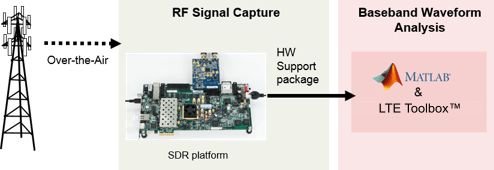In LTE, the broadcast channel (BCH) carries the MIB. The MIB provides basic cell-wide settings, including the system bandwidth and frame number. This example decodes the MIB from a burst of captured frames, and then decodes the control format indicator (CFI) for each subframe, which informs the user equipment (UE) of the control region size. The example then continues to decode the physical downlink control channel (PDCCH). A UE requires these steps to be associated with a cell. For an example showing the procedure for cell search and system information acquisition, see Cell Search, MIB and SIB1 Recovery.

An LTE transmission contains the BCH in the middle six resource blocks (RBs). Therefore, the captured waveform bandwidth needs to be only 1.92 MHz to decode the MIB, regardless of the cell bandwidth. Even though the MIB exists only in subframe 0 of a frame, this example demodulates each entire frame for visualization and analysis.

This example supports these SDRs for LTE waveform capture.

• USRP™ E310/E312 from the Communications Toolbox Support Package for USRP™ Embedded Series Radio

• AD936x/FMCOMMS5 from the Communications Toolbox Support Package for Xilinx® Zynq®-Based Radio

• USRP™ N300/N310/N320/N321/B200/B210/X300/X310 from the Communications Toolbox Support Package for USRP™ Radio

Alternatively, if an SDR is not available for waveform capture, the example supports importing a file with a precaptured waveform.

### Example Setup

Before running the example, ensure that you have installed the appropriate support package for the SDR that you intend to use and that you have set up the hardware.

The `ReceiveOnSDR` field of the `rxsim` structure determines whether the example receives a waveform off the air or imports a waveform from a MAT file.

`rxsim.ReceiveOnSDR =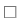false;`

If you use an SDR for reception of LTE waveforms and the SDR detects no LTE waveforms, you can generate and transmit an LTE waveform by using the LTE Transmitter Using Software Defined Radio.

The parameters defined in the `rxsim` structure control the receiver. The sample rate of the receiver is 1.92 MHz, which is the standard sample rate for capturing an LTE bandwidth of 6 RBs. 6 RBs equates to a signal bandwidth of 1.4 MHz. To capture more frames, increase the `rxsim.NumCaptures` parameter value. By default, the example captures five LTE frames with each execution.

Specify the file name of a precaptured waveofrm in the `fileName` variable. Confirm that the MAT file contains a `numCaptures` variable, a `radioSampleRate` variable, and a `capturedData` variable. Each column of `captureData` represents one capture antenna and the third dimension of `captureData` represents the number of captures.

`fileName =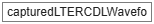"capturedLTERCDLWaveform.mat";`

If you select the `ReceiveOnSDR` field of the `rxsim` structure, configure the variables required for SDR reception. If you use an SDR device capable of multi-channel reception, try increasing `rxsim.NumAntennas` to a value supported by your SDR.

```if rxsim.ReceiveOnSDR rxsim.SDRDeviceName =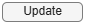"AD936x"; % SDR that is used for waveform reception rxsim.Gain =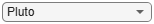45; % Valid values of gain include 'AGC Fast Attack', 'AGC Slow Attack', or an integer value for the Zynq-Based, USRP Embedded, or ADALM-PLUTO radios. USRP radios only support integer values. rxsim.RadioSampleRate =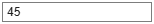1.92e6; % Configured for 1.92e6 Hz capture bandwidth rxsim.RadioCenterFrequency =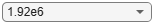806000000; % Center frequency in Hz rxsim.FramesPerCapture =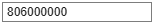8; % Number of contiguous LTE frames to capture rxsim.NumCaptures =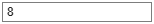1; % Number of captures for the SDR to perform rxsim.NumAntennas =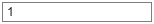1; % Number of receive antennas % Derived parameter captureTime = milliseconds((rxsim.FramesPerCapture + 1)*10); % Increase capture frame by 1 to account for a full frame not being captured else rx = load(fileName); rxsim.NumCaptures = rx.numCaptures; rxsim.RadioSampleRate = rx.radioSampleRate; end```

1. If using an SDR, capture a suitable number of frames of an LTE signal.

2. Determine and correct the frequency offset of the received signal.

3. Determine the cell identity by performing a blind cell search.

4. Synchronize the captured signal to the start of an LTE frame.

5. Extract an LTE resource grid by OFDM demodulating the received signal.

6. Perform a channel estimation for the received signal.

7. Determine the cell-wide settings by decoding the MIB for each captured frame.

8. Decode the CFI and PDCCH for each subframe within the captured signal.

This example plots the power spectral density of the captured waveform and shows visualizations of the received LTE resource grid, estimated channel, and equalized PBCH symbols for each frame.

Configure SDR Hardware

This example communicates with the radio hardware by using the `hSDRReceiver` function to help create an object pertaining to the selected radio. For example, the USRP radios use the `comm.SDRuReceiver` (Communications Toolbox Support Package for USRP Radio) object.

If you are capturing an LTE waveform with an SDR, set the relevant properties in the `sdrReceiver` object with the values configured in the `rxsim` structure.

```if rxsim.ReceiveOnSDR sdrReceiver = hSDRReceiver(rxsim.SDRDeviceName); sdrReceiver.CenterFrequency = rxsim.RadioCenterFrequency; sdrReceiver.SampleRate = rxsim.RadioSampleRate; sdrReceiver.Gain = rxsim.Gain; sdrReceiver.ChannelMapping = 1:rxsim.NumAntennas; end```

Set up the spectrum analyzer to display the received waveform.

```spectrumScope = spectrumAnalyzer( ... SampleRate=rxsim.RadioSampleRate, ... SpectrumType="power-density", ... Title="Baseband LTE Signal Spectrum", ... YLabel="Power Spectral Density");```

LTE Setup

The `enb` structure contains the parameters for decoding the MIB. The example assumes frequency division duplexing (FDD), a normal cyclic prefix length, and four cell-specific reference ports (`CellRefP`) for the MIB decoding as these values are unknown. The MIB provides the actual value of `CellRefP`.

```enb.DuplexMode = "FDD"; enb.CyclicPrefix = "Normal"; enb.CellRefP = 4;```

The sample rate of the signal controls the captured bandwidth and the number of RBs in the waveform. The example uses a lookup table to determine the number of RBs.

```% Bandwidth: {1.4 MHz, 3 MHz, 5 MHz, 10 MHz, 20 MHz} SampleRateLUT = [1.92 3.84 7.68 15.36 30.72]*1e6; NDLRBLUT = [6 15 25 50 100]; enb.NDLRB = NDLRBLUT(SampleRateLUT==rxsim.RadioSampleRate); if rxsim.ReceiveOnSDR fprintf("\nSDR hardware sampling rate configured to capture %d LTE RBs.\n",enb.NDLRB); end```

The `cec` structure controls the channel estimation parameters. To minimize the effect of noise on pilot estimates, use a conservative 9-by-9 pilot averaging window.

```cec.FreqWindow = 9; % Frequency averaging window in resource elements (REs) cec.TimeWindow = 9; % Time averaging window in REs cec.InterpType = "Cubic"; % Cubic interpolation cec.PilotAverage = "UserDefined"; % Pilot averaging method cec.InterpWindow = "Centred"; % Interpolation windowing method cec.InterpWinSize = 3; % Interpolate up to 3 subframes simultaneously```

Signal Capture and Processing

To capture and decode bursts of LTE frames, the example uses a `while` loop. For each captured frame, the example decodes the MIB, and if decoding is successful, it decodes the CFI and the PDCCH for each subframe. For each successfully decoded subframe, the example displays the channel estimate and equalized PDCCH symbols.

Set up the constellation diagram viewer for equalized PDCCH symbols and the figure handle for the channel estimate plots.

```constellation = comm.ConstellationDiagram("Title","Equalized PDCCH Symbols") ; channelEstimatePlot = figure("Visible","Off");```

Store the default decoding parameters.

```enbDefault = enb; samplesPerFrame = 10e-3*rxsim.RadioSampleRate; % LTE frame period is 10 ms```

```for i = 1:rxsim.NumCaptures % Set default LTE parameters enb = enbDefault; % rxWaveform holds |rxsim.FramesPerCapture| number of consecutive % frames worth of contiguous baseband LTE samples. if rxsim.ReceiveOnSDR % SDR Capture fprintf("\nStarting a new RF capture.\n") rxWaveform = capture(sdrReceiver,captureTime); else rxWaveform = rx.capturedData(:,:,i); end % Show power spectral density of captured burst spectrumScope(rxWaveform); release(spectrumScope); % Perform frequency offset correction frequencyOffset = lteFrequencyOffset(enb,rxWaveform); rxWaveform = lteFrequencyCorrect(enb,rxWaveform,frequencyOffset); fprintf("Corrected a frequency offset of %g Hz.\n",frequencyOffset) % Perform the blind cell search to obtain cell identity and timing % offset Use "PostFFT" secondary synchronization signal (SSS) detection % method to improve speed cellSearch.SSSDetection = "PostFFT"; cellSearch.MaxCellCount = 1; [NCellID,frameOffset] = lteCellSearch(enb,rxWaveform,cellSearch); fprintf("Detected a cell identity of %i.\n", NCellID); enb.NCellID = NCellID; % From lteCellSearch % Sync the captured samples to the start of an LTE frame, and trim off % any samples that are part of an incomplete frame. rxWaveform = rxWaveform(frameOffset+1:end,:); tailSamples = mod(length(rxWaveform),samplesPerFrame); rxWaveform = rxWaveform(1:end-tailSamples,:); enb.NSubframe = 0; % OFDM demodulation rxGrid = lteOFDMDemodulate(enb,rxWaveform); % Perform channel estimation [hest,nest] = lteDLChannelEstimate(enb,cec,rxGrid); sfDims = lteResourceGridSize(enb); Lsf = sfDims(2); % OFDM symbols per subframe LFrame = 10*Lsf; % OFDM symbols per frame numFullFrames = length(rxWaveform)/samplesPerFrame; % For each frame, decode the MIB and CFI for frame = 0:(numFullFrames-1) fprintf("\nPerforming MIB decode for frame %i of %i in burst...\n", ... frame+1,numFullFrames) % Extract subframe 0 from each frame of the received resource grid % and channel estimate. enb.NSubframe = 0; rxsf = rxGrid(:,frame*LFrame+(1:Lsf),:); hestsf = hest(:,frame*LFrame+(1:Lsf),:,:); % PBCH demodulation. Extract REs corresponding to the PBCH from the % received grid and channel estimate grid for demodulation. Assume % 4 cell-specific reference signals for PBCH decode as initially % the value is unknown. enb.CellRefP = 4; pbchIndices = ltePBCHIndices(enb); [pbchRx,pbchHest] = lteExtractResources(pbchIndices,rxsf,hestsf); [~,~,nfmod4,mib,CellRefP] = ltePBCHDecode(enb,pbchRx,pbchHest,nest); % If PBCH decoding is not successful, go to next iteration of for- % loop if ~CellRefP fprintf(" No PBCH detected for frame = %d.\n",frame); continue; end % With successful PBCH decoding, decode the MIB and obtain system % information including system bandwidth enb = lteMIB(mib,enb); enb.CellRefP = CellRefP; % From ltePBCHDecode % Incorporate the nfmod4 value output from the function % ltePBCHDecode, as the NFrame value established from the MIB is % the system frame number modulo 4. enb.NFrame = enb.NFrame+nfmod4; fprintf(" Successful MIB Decode.\n") fprintf(" Frame number: %d.\n",enb.NFrame); % The eNodeB transmission bandwidth can be greater than the % captured bandwidth, so limit the bandwidth for processing enb.NDLRB = min(enbDefault.NDLRB,enb.NDLRB); % Process subframes within frame for sf = 0:9 % Extract subframe enb.NSubframe = sf; rxsf = rxGrid(:,frame*LFrame+sf*Lsf+(1:Lsf)); % Perform channel estimation with the correct number of % CellRefP [hestsf,nestsf] = lteDLChannelEstimate(enb,cec,rxsf); % Physical CFI channel (PCFICH) demodulation Extract REs % corresponding to the PCFICH from the received grid and % channel estimate for demodulation. pcfichIndices = ltePCFICHIndices(enb); [pcfichRx,pcfichHest] = lteExtractResources(pcfichIndices,rxsf,hestsf); [cfiBits,recsym] = ltePCFICHDecode(enb,pcfichRx,pcfichHest,nestsf); % CFI decoding enb.CFI = lteCFIDecode(cfiBits); fprintf(" Subframe %d, decoded CFI value: %d.\n",sf,enb.CFI); % PDCCH demodulation. Extract REs corresponding to the PDCCH % from the received grid and channel estimate for demodulation. pdcchIndices = ltePDCCHIndices(enb); [pdcchRx,pdcchHest] = lteExtractResources(pdcchIndices,rxsf,hestsf); [pdcchBits,pdcchEq] = ltePDCCHDecode(enb,pdcchRx,pdcchHest,nestsf); release(constellation); constellation(pdcchEq); end % Plot channel estimate between CellRefP 0 and the receive antenna focalFrameIdx = frame*LFrame+(1:LFrame); figure(channelEstimatePlot); surf(abs(hest(:,focalFrameIdx,1,1))); xlabel("OFDM Symbol Index"); ylabel("Subcarrier Index"); zlabel("Magnitude"); title("Estimate of Channel Magnitude Frequency Response"); end end```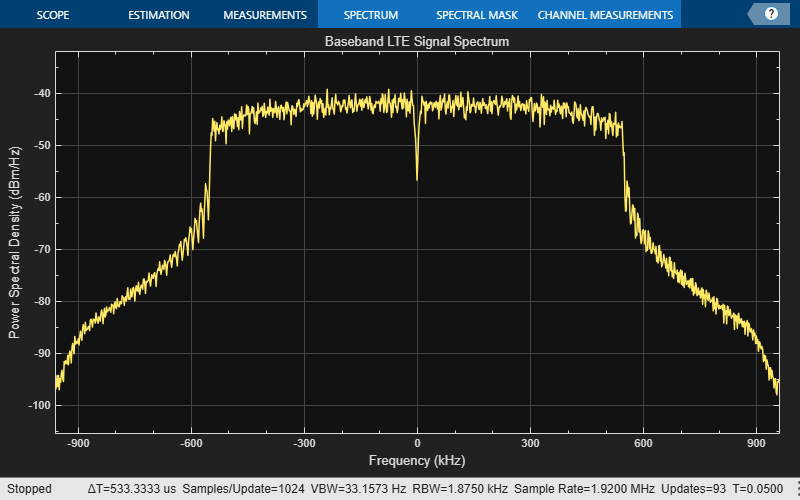```Corrected a frequency offset of -2.48294 Hz. ```
```Detected a cell identity of 17. ```
```Performing MIB decode for frame 1 of 4 in burst... ```
``` Successful MIB Decode. ```
``` Frame number: 705. ```
``` Subframe 0, decoded CFI value: 3. Subframe 1, decoded CFI value: 3. Subframe 2, decoded CFI value: 3. Subframe 3, decoded CFI value: 3. Subframe 4, decoded CFI value: 3. Subframe 5, decoded CFI value: 3. Subframe 6, decoded CFI value: 3. Subframe 7, decoded CFI value: 3. Subframe 8, decoded CFI value: 3. Subframe 9, decoded CFI value: 3. ```
```Performing MIB decode for frame 2 of 4 in burst... ```
``` Successful MIB Decode. ```
``` Frame number: 706. ```
``` Subframe 0, decoded CFI value: 3. Subframe 1, decoded CFI value: 3. Subframe 2, decoded CFI value: 3. Subframe 3, decoded CFI value: 3. Subframe 4, decoded CFI value: 3. Subframe 5, decoded CFI value: 3. Subframe 6, decoded CFI value: 3. Subframe 7, decoded CFI value: 3. Subframe 8, decoded CFI value: 3. Subframe 9, decoded CFI value: 3. ```
```Performing MIB decode for frame 3 of 4 in burst... ```
``` Successful MIB Decode. ```
``` Frame number: 707. ```
``` Subframe 0, decoded CFI value: 3. Subframe 1, decoded CFI value: 3. Subframe 2, decoded CFI value: 3. Subframe 3, decoded CFI value: 3. Subframe 4, decoded CFI value: 3. Subframe 5, decoded CFI value: 3. Subframe 6, decoded CFI value: 3. Subframe 7, decoded CFI value: 3. Subframe 8, decoded CFI value: 3. Subframe 9, decoded CFI value: 3. ```
```Performing MIB decode for frame 4 of 4 in burst... ```
``` Successful MIB Decode. ```
``` Frame number: 700. ```
``` Subframe 0, decoded CFI value: 3. Subframe 1, decoded CFI value: 3. Subframe 2, decoded CFI value: 3. Subframe 3, decoded CFI value: 3. Subframe 4, decoded CFI value: 3. Subframe 5, decoded CFI value: 3. Subframe 6, decoded CFI value: 3. Subframe 7, decoded CFI value: 3. Subframe 8, decoded CFI value: 3. Subframe 9, decoded CFI value: 3. ```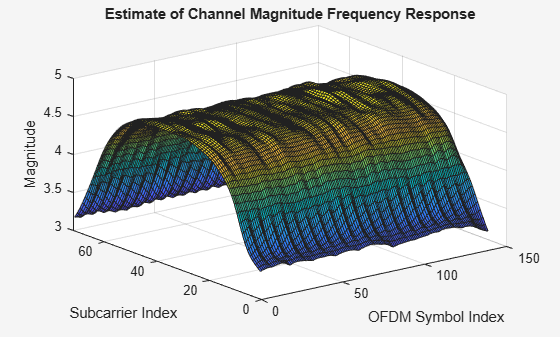```if rxsim.ReceiveOnSDR release(sdrReceiver); end release(constellation); % Release constellation diagram object```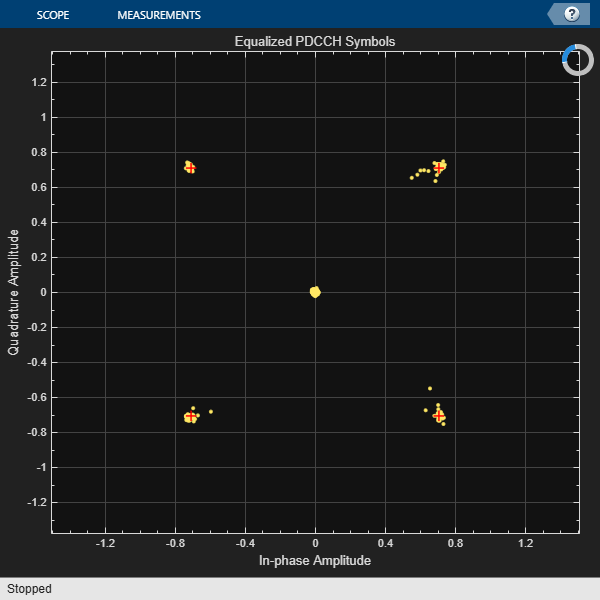### Further Exploration

• Transmit a standard compliant LTE waveform by using the LTE Transmitter Using Software Defined Radio. You can then decode the waveform using this example. In the companion example, try changing the cell identity and initial frame number, and observe the detected cell identity and frame number in this example. If using a supported multi-channel SDR, try increasing the number of antennas in the companion example to visualize the benefit of using multi-channel transmission/reception.

• This example only decodes basic system information. For an example of how to robustly decode more physical channels, see the Cell Search, MIB and SIB1 Recovery example.

### Local Functions

```function waveform = captureWaveform(sdrReceiver,numSamplesToCapture) % CAPTUREWAVEFORM returns a column vector of complex values given an % SDRRECEIVER object and a scalar NUMSAMPLESTOCAPTURE value. % For a comm.SDRuReceiver object, use the burst capture technique to % acquire the waveform if isa(sdrReceiver,'comm.SDRuReceiver') waveform = complex(zeros(numSamplesToCapture,length(sdrReceiver.ChannelMapping))); samplesPerFrame = sdrReceiver.SamplesPerFrame; for i = 1:sdrReceiver.NumFramesInBurst waveform(samplesPerFrame*(i-1)+(1:samplesPerFrame),:) = sdrReceiver(); end else waveform = capture(sdrReceiver,numSamplesToCapture); end end```